Home MonkeyNotes Printable Notes Digital Library Study Guides Study Smart Parents Tips College Planning Test Prep Fun Zone Help / FAQ How to Cite New Title Request

8.3 Concentration of Solution

Concentration of Solutions can be expressed in different ways as follows :

i)Weight percentage of solute is the weight of solute
(´ 100), divided by the total weight of the solution.

i.e. 10% w/w of NaCl means 10 gms of NaCl is present in a 100 g of solution.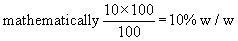ii) The molarity, M, of a solution is the number of moles of solute per kg of solvent.

20 M of sodium chloride solution means 20 moles (20 ´ 55.5 gms) of sodium chloride dissolved in a solvent to get one liter of solution.

iii) The molality, m, of a solution is the number of moles of solute per kg of solution.

12 m of sodium chloride solution means 12 moles (12 ´ 55.5 gms) of sodium chloride dissolved in a solvent to get 1 kg of solution.Your browser does not support the IFRAME tag.
iv) The normality, N, of a solution is the number of gram equivalent weight of solute per liter of solution.

5 N of sulphuric acid solution means ( 5 ´ 49 gms ) ( \ eq. wt = mol.wt / no.of equivalents = 98/2 = 49 ) of sulphuric acid dissolved in solvent to get one liter of solution.

v) The mole fraction, X, is the ratio of the number of moles of solute ( or solvent ) to the total number of moles of all components in a solution.

In a 10% HCl solution in H2O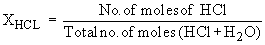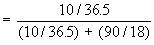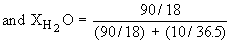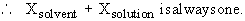At a glance the concentration of solutions:

 No. Name Symbol Explanation 1 Weight percentage % w/w Weight of solute in percentage in 100 gm of solution. 2 Molarity M Number of moles of solute per liter of solution. 3 Molality m Number of moles of solute per kg of solution. 4 Normality N Number of gram equivalent weights of solute per liter of solution. 5 Mole fraction X Ratio of the number of moles of solute (or solvent) to the total number of moles of all components in a solution.

Index

8.1 Nature of Solution
8.2 Heat of Solutions
8.3 Concentration of Solution
8.4 Effect of Temperature and Pressure on Solubility
8.5 Boiling Point and Freezing Point of Solution

Chapter 9Search: All Products Books Popular Music Classical Music Video DVD Toys & Games Electronics Software Tools & Hardware Outdoor Living Kitchen & Housewares Camera & Photo Cell Phones Keywords: